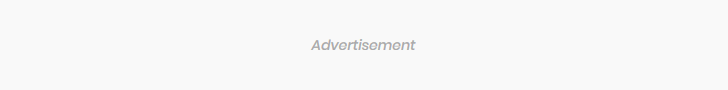### Subscribe Us# Differentation (CIE)

$\def\D{\displaystyle}$

1 (CIE 2012, s, paper 12, question 5)
Differentiate the following with respect to $\D x.$
(i) $\D (2-x^2)\ln (3x+1)$ 
(ii) $\D \frac{4-\tan 2x}{5x}$ 

2 (CIE 2012, s, paper 21, question 7)
Given that $\D f(x)=x^2-\frac{648}{\sqrt{x}},$ find the value of $\D x$ for which $\D f''(x)=0.$ 

3 (CIE 2012, w, paper 11, question 5)
Given that $\D y=\frac{x^2}{\cos 4x},$ find
(i) $\D \frac{dy}{dx}$, 
(ii) the approximate change in $\D y$ when $\D x$ increases from $\D \frac{\pi}{4}$to $\D \frac{\pi}{4}+p$, where $\D p$ is small. 

4 (CIE 2013, s, paper 22, question 7)
Differentiate with respect to $\D x$,
(i) $\D (3-5x)^{12}$ 
(ii)$\D x^2\sin x$ 
(iii)$\D \frac{\tan x}{1+e^{2x}}$ 

5 (CIE 2014, s, paper 21, question 7)
Given that a curve has equation $\displaystyle y=\frac{1}{x}+2\sqrt{x},$ where $\D x>0,$ find

(i) $\D \frac{dt}{dx},$ 
(ii) $\D \frac{d^2y}{dx^2}.$ 

Hence, or otherwise, find
(iii) the coordinates and nature of the stationary point of the curve.


6 (CIE 2014, s, paper 21, question 10)
Find $\D \frac{dy}{dx}$ when
(i) $\D y=\cos 2x \sin\left(\frac{x}{3}\right),$ 
(ii) $\D y=\frac{\tan x}{1+\ln x}.$

7 (CIE 2014, s, paper 22, question 7)
Differentiate with respect to $\D x$
(i) $\D x^4e^{3x},$ 
(ii) $\D \ln(2+\cos x),$ 
(iii) $\D \frac{\sin x}{1+\sqrt{x}}.$ 

8 (CIE 2015, w, paper 23, question 3)
(a) Given that $\D y=\frac{x^3}{2-x^2},$ find $\D \frac{dy}{dx}.$ 
(b) Given that $\D y=x\sqrt{4x+6},$ show that $\D \frac{dy}{dx}= \frac{k(x+1)}{\sqrt{4x+6}}$ and state the value of $\D k.$ 

9 (CIE 2016, march, paper 22, question 1)
Two variables $\D x$ and $\D y$ are such that $\D y=\frac{5}{\sqrt{x-9}}$ for $\D x>9$.
(i) Find an expression for $\D \frac{dy}{dx}.$ 
(ii) Hence, find the approximate change in $\D y$ as $\D x$ increases from 13 to $\D 13+h$, where $\D h$ is small. 

10 (CIE 2016, s, paper 12, question 6)
Show that $\D \frac{d}{dx}(e^{3x} \sqrt{4x+1})$ can be written in the form $\D \frac{e^{3x}(px+q)}{\sqrt{4x+1}},$ where $p$ and $q$ are integers to be found. 

11 (CIE 2017, s, paper 11, question 10)
(a) Given that $\D y=\frac{e^{3x}}{4x^2+1},$ find $\D \frac{dy}{dx}.$ 
(b) Variables $x,y,$ and $t$ are such that $\D y=4\cos \left(x+\frac{\pi}{3}\right) + 2\sqrt{3}\sin\left(x+\frac{\pi}{3}\right)$ and $\D \frac{dy}{dt}=10.$
(i) Find the value of $\D \frac{dy}{dx}$ when $\D x=\frac{\pi}{2}.$ 
(ii) Find the value of $\D\frac{dx}{dt}$ when $\D x=\frac{\pi}{2}.$ 

12 (CIE 2017, s, paper 12, question 2)
It is given that $\D \frac{(5x^2+4)^{\frac{1}{2}}}{x+1}.$
Showing all your working, find the exact value of $\D \frac{dy}{dx}$
when $\D x = 3.$


13 (CIE 2017, s, paper 21, question 3)
The variables $\D x$ and $\D y$ are such that $\D y = \ln(x^2 + 1).$
(i) Find an expression for $\D \frac{dy}{dx}.$

(ii) Hence, find the approximate change in $\D y$ when $\D x$ increases from 3 to $\D 3 + h,$ where $h$ is small. 

14 (CIE 2017, s, paper 23, question 7)
Differentiate with respect to $\D x$.
(i) $\D (1+4x)^{10}\cos x,$
(ii) $\D\frac{e^{4x-5}}{\tan x}.$

15 (CIE 2017, w, paper 12, question 4)
Given that $\D y= \frac{\ln(3x^2+2)}{x^2+1},$
find the value of $\D \frac{dy}{dx}$
when $\D x = 2,$ giving your answer as $\D a + b \ln 14,$ where
$a$ and $b$ are fractions in their simplest form. 

1.(i) $\D\frac{3(2-x^2)}{3x+1}-2x\ln(3x+1)$
(ii) $\D \frac{-10x\sec^22x+5\tan2x-20}{25x^2}$
2. $\D x=9$
3. (i) $\D \frac{2x\cos4x+4x^2\sin4x}{\cos^24x}$
(ii) $\D \frac{-\pi}{2}p$
4. (i) $\D -60(3-5x)^{11}$
(ii) $\D x^2\cos x+2x\sin x$

(iii)$\D \frac{(1+e^{2x})\sec^2x-\tan x(2e^{2x})}{(1+e^{2x})^2}$
5. (i)$\D \frac{-1}{x^2}+\frac{1}{\sqrt{x}}$
(ii)$\D \frac{2}{x^3}-\frac{1}{2x^{\frac{3}{2}}}$
(iii) $\D (1,3)$, min
6. (i) $\D \cos\frac{x}{3}\frac{\cos2x}{3} -2\sin(2x)\sin(\frac{x}{3})$
(ii) $\D \frac{(1+\ln x)\sec^2x-\frac{\tan x}{x}}{(1+\ln x)^2}$
7. (i) $\D 4x^3e^{3x}+ 3x^4e^{3x}$
(ii) $\D \frac{-\sin x}{2+\cos x}$
(iii) $\frac{(1+\sqrt{x})\cos x-\sin x(\frac{1}{2\sqrt{x}})}{(1+\sqrt{x})^2}$
8. (a)$\D \frac{(2-x^2)3x^2+2x^4}{(2-x^2)^2}$
(b) $\D k=6$
9. (i) $\D\frac{-5}{2(x-9)^{3/2}}$
(ii) $\D -0.3125h$
10. $\D p=12,q=5$
11. (i) (a) $\D \frac{3e^{3x}}{4x^2+1}- \frac{8xe^{3x}}{(4x^2+1)^2}$
(b) $\D -5,-2$
12. 11/112
13 (i) $\D \frac{2x}{x^2+1}$
(ii) $\D 0.6 h$
14(i) $\D (1+4x)^{10}(-\sin x)+40 (1+4x)^9\cos x$
(ii) $\D \frac{e^{4x-5}(4\tan x)-\sec^2x}{\tan x}$
15. $\D \frac{6}{35}-\frac{4}{25}\ln 14$